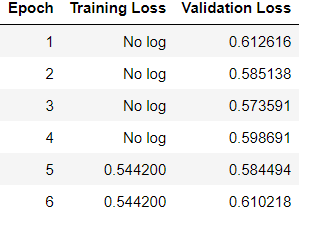# TokenClassification with Bert does not seem to learn at all

Hey guys,

I have a project where I need to classify a sequence of tokens in a given sentence as either 0: unrelevant or 1: relevant. I tried to follow the tutorial for TokenClassification with DestillBert/Bert from Huggingface for NER, but the transfer does not seem to result in a model that learns to make predictions. The Bert model from the original paper and data seems to work fine and is able to make predictions on new data. As a side-node: I changed the original topic-based (think Topic 1 and Topic 2 for training and Topic 3 for testing) split to 90 / 10 over all topic, but I expect the problem to lay in the program logic rather than in the data split. Thanks for taking your time, if you are reading this!

Data is of the following format:

tokens: List(String) [‘I’, ‘am’ ‘an’ ‘example’ , ‘.’ ]
labels: List(Integer) [0, 1, 1, 1, 0]

This is the Dataset format I use. As the tokens from the dataset were extracted with Bert, I just convert them to their IDs and stitch them together with the special tokens [CLS] and [SEP]. For the special tokens I assign a label of -100 to ignore them during the loss computation.

``````from torch.utils.data import Dataset

class relDataset(Dataset):

def __init__(self, tokens, labels, tokenizer):
self.tokens = tokens
self.labels = labels
self.tokenizer = tokenizer

def __getitem__(self, idx):

encoding = {}
encoding["input_ids"] =  + tokenizer.convert_tokens_to_ids(self.tokens[idx]) + 
encoding["labels"] = [-100] + self.labels[idx] + [-100]

return encoding

def __len__(self):
return len(self.label)
``````
``````train = rel_Dataset(tokens = train_df["tokens"].values,
labels = train_df["labels"].values,
tokenizer = tokenizer)
``````
``````test = rel_Dataset(tokens = test_df["tokens"].values,
labels = test_df["labels"].values,
tokenizer = tokenizer)
``````

Then I set up tokenizer, model, and trainer

``````from transformers import AutoTokenizer
from transformers import AutoModelForTokenClassification, TrainingArguments, Trainer
from torch.nn.parallel import DataParallel
import torch

tokenizer = AutoTokenizer.from_pretrained("distilbert-base-uncased")
model = AutoModelForTokenClassification.from_pretrained("distilbert-base-uncased", num_labels=2)
device = torch.device("cuda")
model.to(device)

training_args = TrainingArguments(
evaluation_strategy="epoch",
learning_rate=2e-5,
per_device_train_batch_size=32,
per_device_eval_batch_size=32,
num_train_epochs=6,
weight_decay=0.01
)

trainer = Trainer(
model=model,
args=training_args,
train_dataset=train_A8,
eval_dataset=eval_A8,
tokenizer=tokenizer,
data_collator=data_collator,
#compute_metrics=compute_metrics
)
``````

Then using Trainer to train the model I achieve the following performance
trainer.train()The Training loss seems to be static, while the predictions on the test data do not seem to make a difference